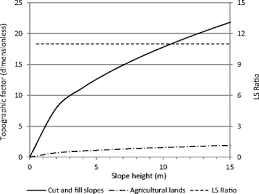## How to Calculate and Solve for Universal Soil Loss | Water BudgetThe image above represents universal soil loss.

To compute for universal soil loss, six essential parameters are needed and these parameters are Rainfall and Run off Activity Index by Geological Location (R), Soil Erodibility Factor (K), Topographic Factor (LS), Yield Slope (S), Cropping Management Factor (C) and Conservation Practice Factor (P).

The formula for calculating universal soil loss:

A = 2.24(R)(K) . (LS) . (C)(P)

Where:

A = Universal Soil Loss
R = Rainfall and Run off Activity Index by Geological Location
K = Soil Erodibility Factor
LS = Topographic Factor
S = Yield Slope
C = Cropping Management Factor
P = Conservation Practice Factor

Let’s solve an example;
Find the universal soil loss when the rainfall and run off activity index by geological location is 12, the soil erodibility factor is 8, the topographic factor is 9, yield scope is 4, cropping management factor is 2 and the conservation practice factor is 7.

This implies that;

R = Rainfall and Run off Activity Index by Geological Location = 12
K = Soil Erodibility Factor = 8
LS = Topographic Factor = 9
S = Yield Slope = 4
C = Cropping Management Factor = 2
P = Conservation Practice Factor = 7

A = 2.24(R)(K) . (LS) . (C)(P)
A = 2.24(12)(8) . (9) . (2)(7)
A = 2.24(12096)
A = 27095.04

Therefore, the universal soil loss is 27095.04.

Calculating for Rainfall and Run off Activity Index by Geological Location when the Universal Soil Loss, the Soil Erodibility Factor, the Topographic Factor, the Cropping Management Factor and the Conservation Practice Factor is Given.

R = A / 2.24(K) (LS) C.P

Where:

R = Rainfall and Run off Activity Index by Geological Location
A = Universal Soil Loss
K = Soil Erodibility Factor
LS = Topographic Factor
C = Cropping Management Factor
P = Conservation Practice Factor

Let’s solve an example;
Find the rainfall and run off activity index by geological location when the universal soil loss is 12, the soil erodibility factor is 8, the topographic factor is 10, the cropping management factor is 4 and the conservation practice factor is 7.

This implies that;

A = Universal Soil Loss = 12
K = Soil Erodibility Factor = 8
LS = Topographic Factor = 10
C = Cropping Management Factor = 4
P = Conservation Practice Factor = 7

R = A / 2.24(K) (LS) C.P
R = 12 / 2.24(8) (10) (4)(7)
R = 12 / 2.24(8) (10) (4)(7)
R = 12 / 2.24(2240)
R = 12 / 5017.6
R = 0.00239

Therefore, the Rainfall and run off activity index by geological location is 0.00239.

Calculating for Soil Erodibility Factor when the Universal Soil Loss, the Rainfall and Run off Activity Index by Geological Location, the Topographic Factor, the Cropping Management Factor and the Conservation Practice Factor is Given.

K = A / 2.24(R) (LS) C.P

Where:

K = Soil Erodibility Factor
R = Rainfall and Run off Activity Index by Geological Location
A = Universal Soil Loss
LS = Topographic Factor
C = Cropping Management Factor
P = Conservation Practice Factor

Let’s solve an example;
Find the soil erodibility factor when the rainfall and run off activity index by geological location is 20, the universal soil loss is 15, the topographic factor is 2, the cropping management factor is 9 and the conservation practice factor is 6.

This implies that;

R = Rainfall and Run off Activity Index by Geological Location = 20
A = Universal Soil Loss = 15
LS = Topographic Factor = 2
C = Cropping Management Factor = 9
P = Conservation Practice Factor = 6

K = A / 2.24(R) (LS) C.P
K = 15 / 2.24(20) (2) (9)(6)
K = 15 / 4838.4
K = 0.0031

Therefore, the soil erodibility factor is 0.0031.
Continue reading How to Calculate and Solve for Universal Soil Loss | Water Budget